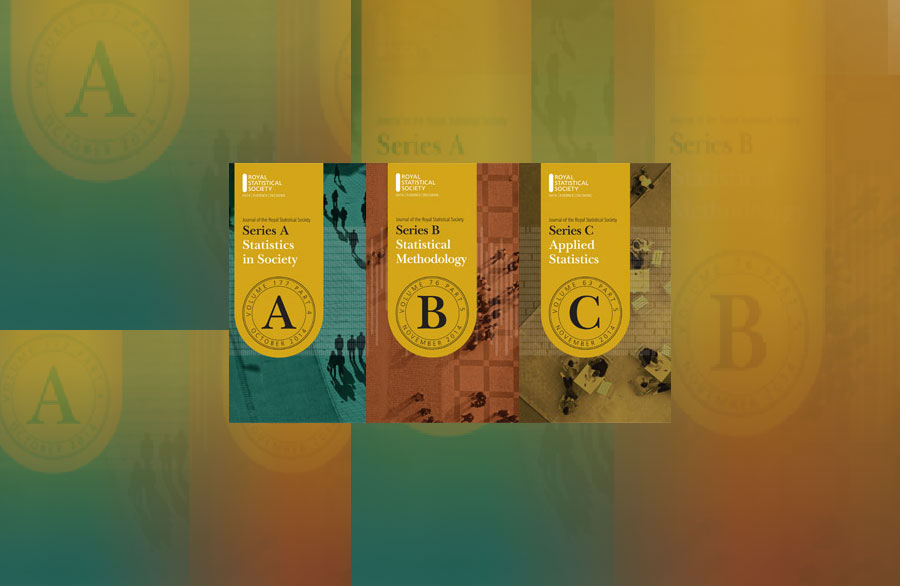# RSS journals all increase Impact Factor scores for 2020

The 2020 Impact Factors have just been released. The score for all three RSS Journals – Series A, B and C – have increased compared to 2019.

Series A is now 2.483 (was 2.210) with a five-year impact factor of 3.233. The journal is now ranked 28/125 (statistics & probability) and 18/52 (social sciences, mathematical methods).

Series B is now 4.488 (was 3.965) with a five-year impact factor of 5.701. The journal is now ranked 10/125 (statistics & probability)

Series C is now 1.864 (was 1.590) with a five-year impact factor of 2.179. The journal is now ranked 51/125 (statistics & probability).

Congratulations to all of those involved in producing the Journals!